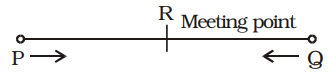Home » Aptitude » Speed, Time and Distance » Question

#### Speed, Time and Distance

1. Two places P and Q are 162 km apart. A train leaves P for Q and simultaneously another train leaves Q for P. They meet at the end of 6 hours. If the former train travels 8 km/hour faster than the other, then speed of train from Q is
1.  12 5 km/hour 6
2.  10 5 km/hour 6
3.  9 1 km/hour 2
4.  8 1 km/hour 2
##### Correct Option: CSpeed of train starting from Q = x kmph
∴  Speed of train starting from P = (x + 8) kmph
According to the question,
PR + RQ = PQ
⇒  (x + 8) × 6 + x × 6 = 162
[Distance = Speed × Time]
⇒  6x + 48 + 6x = 162
⇒  12x = 162 – 48 = 114

 ⇒  x = 114 = 19 12 2

 = 9 1 kmph 2